Email us to get an instant 20% discount on highly effective K-12 Math & English kwizNET Programs!

#### Online Quiz (WorksheetABCD)

Questions Per Quiz = 2 4 6 8 10

### Grade 5 - Mathematics5.2 Types of Fractions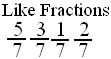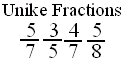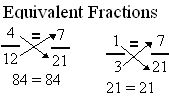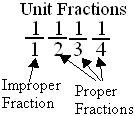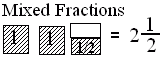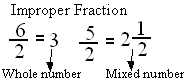Directions: Answer the following questions. Also write at least 5 examples of each type of fractions.
 Q 1: If the numerator is more than the denominator in a fraction, the fraction is called a _________.mixed fractionimproper fractionproper fraction Q 2: A mixed number denotes the sum of a whole number-part and proper fraction part.FalseTrue Q 3: If the numerator is less than the denominator in a fraction, the fraction is called a _______.improper fractionmixed fractionproper fraction Q 4: Fraction with numerator 1 is called a unit fraction. Are all unit fractions proper?YesNo Q 5: A number with a whole number and a proper fraction is amixed numberproper number Q 6: Fractions with different denominators are calledunlike fractionslike fractions Q 7: When a products obtained by cross multiplication give the same number, then the fractions are ____(or equal).mixedproperequivalent Q 8: An improper fraction is sometimes a mixed number or sometimes a whole number.FalseTrue Question 9: This question is available to subscribers only! Question 10: This question is available to subscribers only!